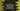# C program to print the multiplication table using do...while loop## C program to print the multiplication table using do…while loop:

In this post, we will learn how to print the multiplication table using do…while loop in C. The program will take a number as input from the user and print the multiplication table for that number. With this program, you will learn how to use do…while loops in C, how to read user inputs and how to print values on the console.

### do…while loop:

The do…while loop has the following syntax:

``````do{
// body
}while(condition);``````

The do…while loop first executes the statements of its body and then it checks the condition which is written in the while block. So, even if the condition is false, it will execute the body.

### Method 1: C program to print the multiplication table with a do…while loop:

Let’s use a do…while loop to print the multiplication table:

``````#include <stdio.h>

int main()
{
int no, i = 1;
scanf("%d", &no);

do
{
printf("%d * %d = %d\n", no, i, no * i);
i++;
} while (i <= 10);

return 0;
}``````

In this program,

• The integer no is used to hold the user input number. Another integer variable i is initialized as 1 to use in the loop.
• It asks the user to enter the number. It reads it by using scanf and store it in the no variable.
• The condition in the do-while loop is i <= 10 i.e. it will run till the value of i is smaller than or equal to 10.
• On each iteration, we are printing one step of the multiplication table. At the end of each iteration, the value of i is incremented by 1.

Sample output:

``````Please enter a number: 5
5 * 1 = 5
5 * 2 = 10
5 * 3 = 15
5 * 4 = 20
5 * 5 = 25
5 * 6 = 30
5 * 7 = 35
5 * 8 = 40
5 * 9 = 45
5 * 10 = 50

9 * 1 = 9
9 * 2 = 18
9 * 3 = 27
9 * 4 = 36
9 * 5 = 45
9 * 6 = 54
9 * 7 = 63
9 * 8 = 72
9 * 9 = 81
9 * 10 = 90``````

### Method 2: C program to print the multiplication table up to a given limit:

In the above example, the multiplication table is printed from 1 to 10 for a given number. We can also take the limit as input from the user and print the table from 1 to the given limit. Let’s change the above program to use a limit to print the table:

``````#include <stdio.h>

int main()
{
int no, i = 1, limit;

scanf("%d", &no);

printf("Enter the limit: ");
scanf("%d", &limit);

do
{
printf("%d * %d = %d\n", no, i, no * i);
i++;
} while (i <= limit);

return 0;
}``````

Here, we added one more integer variable limit to store the limit. The do…while loop will run till this value. Let’s take a look at the below example:

``````Please enter a number: 11
Enter the limit: 12
11 * 1 = 11
11 * 2 = 22
11 * 3 = 33
11 * 4 = 44
11 * 5 = 55
11 * 6 = 66
11 * 7 = 77
11 * 8 = 88
11 * 9 = 99
11 * 10 = 110
11 * 11 = 121
11 * 12 = 132``````

The number is 11 and the limit is 12. So, it will print the multiplication table from 1 to 12 for 11.

### Method 3: C program to print the multiplication table by using a separate function:

Let’s use a separate function to print the multiplication table. This function will take the number as the parameter and print the multiplication table for that number.

``````#include <stdio.h>

void printMultiplicationTable(int no)
{
int i = 1;
do
{
printf("%d * %d = %d\n", no, i, no * i);
i++;
} while (i <= 10);
}

int main()
{
int no;